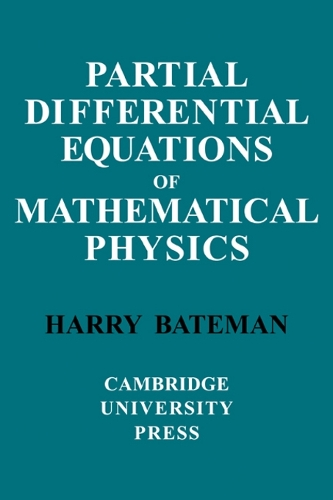•# Partial Differential Equations of Mathematical Physics (Paperback)

(author)
£40.99
Paperback 548 Pages / Published: 01/12/1932
• We can order this

Usually dispatched within 3 weeks

Harry Bateman (1882-1946) was an esteemed mathematician particularly known for his work on special functions and partial differential equations. This book, first published in 1932, has been reprinted many times and is a classic example of Bateman's work. Partial Differential Equations of Mathematical Physics was developed chiefly with the aim of obtaining exact analytical expressions for the solution of the boundary problems of mathematical physics.

Publisher: Cambridge University Press
ISBN: 9780521091633
Number of pages: 548
Weight: 800 g
Dimensions: 229 x 152 x 31 mm